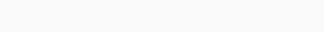# Lemmatization with TextBlob in Python

In some processes of text analyzation lemmatization used. Lemmatization is one form of NLP. It used for extracting the high quality of information from text data. Now this Lemmatization in Python by using Textblob explains as follow:

## Lemmatization

The process of converting the word to its base form is lemmatization. Lemmatization is closely related to stemming but it is more accurate than stemming. Stemming can lead to incorrect spelling and wrong meanings, but lemmatization gives a correct base form of a word. Lemmatization would correctly identify the word in a single item called a lemma. Lemmatization is an algorithmic way to determine the word or lemma. Stemming just remove some characters from a word. If some word has more than one lemma then lemmatization correctly identifies the base word based on context.

For example- lemmatization correctly identify ‘sharing’ to ‘share’. But stemming just removes ‘ing’ from word and makes it ‘shar’.

‘Sharing’ -> Lemmatization -> ‘Share’

‘Sharing’ -> Stemming -> ‘Shar’

## TextBlob

TextBlob is a Python library used to perform some basic tasks of NLP. For example part of speech tagging, sentiment analysis, tokenization, lemmatization, etc.

You can install it by command: pip install TextBlob

Here, we use the Python library TextBlob for lemmatization.

## Implementation of Lemmatization with TextBlob in Python

Importing Textblob library.

`from textblob import TextBlob,Word`

Lemmatization of word ‘share’.

```sh=Word("sharing")
print("Lemmatization of sharing: ",sh.lemmatize("v"))```

Output:Lemmatization of the sentence ‘You are playing better than me’. When all word lemmatizes for noun paying give play.

```sentence="you are playing better than me"
w=sentence.split(" ")
print(w)
print([Word(word).lemmatize() for word in w])
print([Word(word).lemmatize("v") for word in w])```

Output:```b=Word("better")
#Verb
print(b.lemmatize("v"))
print(b.lemmatize("a"))
#Noun
print(b.lemmatize("n"))
#Abverb
print(b.lemmatize("r"))```

Output:#### Conclusion

Here, we learn the followings:

• Lemmatization
• TextBlob
• Implementation of lemmatization in Python